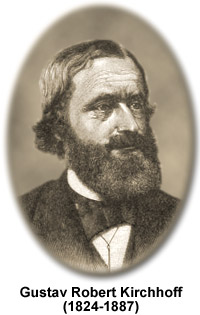Objective:

• To be able to describe Kirchhoff’s first law and appreciate that this is a consequence of conservation of charge.

Who was Kirchhoff?Gustav Robert Kirchhoff was a German physicist who contributed significantly to the understanding of electrical circuits. Here we will be focussing on his first law focussing on circuitry.

Kirchhoff’s First Law, KFL

Kirchhoff’s first law states that the total current entering a junction is equal to the total current leaving that same junction.

Kirchhoff’s first law is a statement of conservation of charge as it means that the total charge flowing into a junction in a given time is equal to the total charge leaving the junction in the same time. Electrons do not jump out a wire or disappear, therefore all the electrons entering one junction must also leave that same point.

We can write the law in the following formats:

The sum of the currents entering a junction = the some of the currents leaving the junction$\Sigma I_{in} = \Sigma I_{out}$

The equations above take into account he direction that the conventional current is flowing in. If you take the following scenario;$I_{1} + I_{2} + I_{3} + I_{4} = 0$

Although this may seem odd, the direction of current is not clear. Lets say that both the current in$I_{1}$ and$I_{3}$ are to the right. Since$I_{1}$ is entering the junction and$I_{3}$ is exiting the junction, it should be shown that$I_{3}$ is a ‘negative current’. If we assume that$I_{2}$ and$I_{4}$ are entering the junction then the above equation should really be written as;$I_{1} + I_{2} + -I_{3} + I_{4} = 0$

And so,$I_{1} + I_{2} + I_{4} = I_{3}$

This equation then shows us that all the current entering the junction must leave through the branch labelled$I_{3}$ which is perfectly acceptable.

Electrons, a form of matter, travel down$I_{1}$$I_{2}$ and$I_{4}$, since matter does not just get destroyed or disappear, it instead comes out and travels down$I_{3}$.

Here are some questions for you to practice applying Kirchhoff’s first law to – Kirchhoff’s 1st Law questions.

Although this law may seem simplistic and may make perfect sense, it is a fundamental building block to understanding what goes on inside electrical circuits.